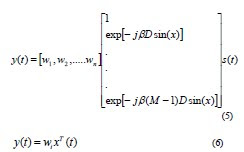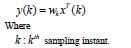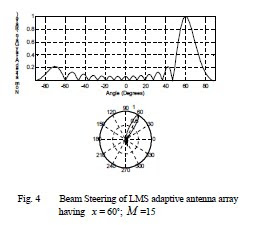## lunes, 26 de julio de 2010

### Adaptive Antenna Array for Satellite

Antennas are classified as either single element or array antennas (multi element). Single element antennas are either omni directional or directional. Omni directional antennas have equal gain in all directions and are also known as isotropic antennas. Directional antennas have maximum gain in desired directions and less in others.

Antenna array is an arrangement of many individual antennas which are placed in space and phase such that the contribution of individual antennas add in one desired direction and cancel in other directions. The antenna arrays are used to generate electronically steer able antenna patterns. The adaptive antenna array adjusts their pattern automatically to signal environment to reduce interference. The desired signal reception is maintained by steering the main beam. Spacing between array elements is an important factor in designing antenna arrays.

Antenna array may be phased array or adaptive array. A phased array antenna system is an array of omni directional or directional element antennas in which the signal induced on the antennas are combined to form an array output. Such an antenna system controls the direction where maximum gain appears by adjusting the phase difference between different antenna elements. An adaptive antenna array combines the outputs of antenna elements but controls the directional gain of the antenna by adjusting both phase and amplitude of the signal at each individual element. Satellite antenna consists of a parabolic reflector with horn antenna at its focus. Basically horn is used as feed for parabolic reflector. Usually Horn is placed at the focus of paraboloid.. The horn antenna have desirable property that it effects a smooth transition from medium like waveguide which supports finite number of propagating modes to a medium like free space which supports a infinite number of modes.In most satellite communication systems, interference remains a problem for reliable reception of signals. Adaptive antenna array for satellite communication systems is better than omni directional antenna that radiate in all directions. Hence for this we use adaptive antenna array that automatically steer the beam in the direction of desired signal i.e. signal of interest (SOI). An antenna array has same directional performance as that of larger antenna . And radiation pattern is an important property of antenna. The overall radiation pattern of an antenna array is obtained by the radiation pattern of the individual elements, their positions, orientation in space and relative phase and amplitudes of the feeding currents to the elements.

Adaptive antenna arrays have ability to adapt changing environment conditions to maximize signal strength of signal of interest (SOI) . In this paper MATLAB software has been used to obtain array voltage patterns for different values of number of elements in antenna array i.e. M and angle of beam steering i.e. x by using LMS algorithm.

ARRAY DESIGN ARCHITECTURE
The overall array pattern can be steered in direction of desired user without physically moving any of individual elements by varying amplitude and phase of individual elements output before combining . The overall radiation pattern of an array is obtained by radiation pattern of individual elements, their positions, orientation in space and the relative amplitude and phase of feeding currents to the elements .Figure '1'shows adaptive antenna array whose architecture is based on LMS algorithm .It is shown in the figure that antenna array is a linear array in which centers of antenna elements are placed along straight line. Since the signals incident on all the antenna elements are of different phases due to the difference in distance traveled by the wave between two antenna elements.

The signal present at element one has traveled more distance Dsin( x) than signal present at element two .Where D is distance between successive antenna phase centers in the array as shown in figure 1. Therefore phase of element one will lag behind that of element two by βDsin(x).
Where

β (Phase propagation factor)= 2π /λ

Here λ =wavelength of received signal. In this figure1 the incident waves are defined as s(t) . We will assume that the receiver will down convert the signal to its Intermediate Frequency (IF) while the Analog-to-Digital converter (A/ D) will down convert the signal to its base band equivalent . As they reach the antenna elements, the waves are converted to electrical sinals x(t) . From Figure1, we define the input signals as ( ), ( ),......... ( ) 1 2 x t x t x t n . These signals are then multiplied by the input weights n w ,w ,........w 1 2 . Then output signal y(t) is the weighted sum of the input signalsWhere
n : Number of the weights. The error signal e(k) in the following figure represents the difference between the summed output, y(k) and the reference signal r(k) . The error processor then computes the required weight adjustment necessary in order to null out the undesired signal. This process is an iterative process and will continue until all the weights in the array converge. Consider a narrow band incident wave as:

S(t) = Aexp(2πf t +φ ) c (2)

Where
A : Amplitude of the signal.
c f : Carrier frequency.
φ : Phase difference between incidents waves at
successive elements. i.e. φ = 2π /λDsin(x)By taking received signal at element one as the reference, the received signals x (t) i for uniform linear array with element spacing D is represented in matrix form as.For adaptive beam forming, each element output x (t) i is multiplied with weight i w that modify phase and amplitude relation between the branches and summed to give output y(t).The overall antenna pattern is continuously modified by adjusting weight vector. For digital communication system, the input signals are in discrete time sampled data form.

Therefore output is:LMS ALGORITHM
Basic Description

The LMS algorithm was first introduced by Widrow et al and operates with a priori knowledge of the direction of arrival and the spectrum of the signal but with no knowledge of the noise and interference in the channel. This algorithm is useful when the interference contains some pectral correlation with the SOI. Minimization of the MMSE can be accomplished by a gradient-search technique. The particular method that the LMS algorithm uses is known as the steepest decent technique. For this particular technique, the changesin the weight vector are made along the direction of the estimated gradient vector.Here we can see that the LMS algorithm does not require squaring, averaging or differentiating and hence can be implemented in most practical systems . Its popularity is accredited to the fact that it is simple, easy to compute and efficient. However, the drawback is that the weights for this algorithm take a long time to converge.

B. The Convergence Rate of the LMS Algorithm The parameter μ is the gain constant that regulates the speed and stability of adaptation. It determines the convergence rate. This gain factor is bounded by the limits

0 < μ <>
Where max λ : Largest Eigen value of the input correlation matrix. RESULTS Figures 2, 3, 4 show array voltage patterns for different values of number of elements in antenna array (M) and angle of beam steering (x) as given in table I.The magnitude of the initial pattern is determined by the initial (arbitrary) choice of weights, w whereas the magnitude of the final pattern is determined by the strength of the desired signal, the direction and strength of the interfering signal and the noise in the system.

It is observed in figure 2, maximum gain occurs at an angle of 30° as angle of beam steering is fixed at 30°. The figure 3 shows that main beam is at an angle of 45° because signal is received by the receiver at this angle In figure 4, the maximum gain occurs at an angle of 60° as angle of beam steering is fixed at 60°Jorge Polentino

CRF

http://www.iaeng.org/publication/IMECS2008/IMECS2008_pp1491-1494.pdf